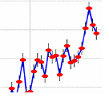Drought and immunity determine the intensity of West Nile virus epidemics and climate change impacts Figure 2.Climate and immunity correlations with annual state WNND cases.The effect of (a) immunity (cumulative incidence; coeff. = -2.05, F1,300 = 96.42, p < 0.001), (b) precipitation (coeff. = -0.0009, F1,161 = 2.20, p = 0.14), (c) drought (coeff. = -0.14, F1,274 = 27.01, p < 0.001), (d) winter severity (coeff. = -0.05, F1,34 = 2.95, p = 0.09), (e) temperature (PIP: coeff. = 0.06, F1,276 = 2.58, p = 0.10; TAR: coeff. = 0.22, F1,144 = 53.59, p < 0.001; QUI: coeff. = 0.002, F1,104 = 0.0005, p = 0.98) and (f) temperature modelled as the relative R0 value at a given temperature (coeff. = 1.66, F1,121 = 17.33, p < 0.001) on the total logged number of WNND cases (adjusted for state random effects) in a given state and year (1999–2013). In (a–d,f), the filled red points and fitted lines are univariate regressions for states in which that predictor was significant (a < 0.05), while open black points depict states in which the predictor was not significant. In (e), green crosses, blue circles and green triangles denote states where C. tarsalis, C. pipiens and C. quinquefasciatus, respectively, dominate transmission and the relationship is only significant for C. tarsalis. (Online version in colour.) URL:     http://carbonbrief.us2.list-manage.com/track/click?u=39b25e6afa81d7ffc0e925ee9&id=ad7e6061a2&e=bf36e91829